# Area of Triangle

Area of a triangle is the region enclosed by these three sides of the triangle i.e. it can be considered as the space occupied by the boundaries of the triangle. We can calculate the area of triangle if the base and height of the triangle are known. Hence, the area of triangle can be calculated by the formula half of the product of Base and Height. Area of triangle is measured in square units and the SI unit of area is sq. m or m2.

In this article, we will learn about what is area of a triangle, area of equilateral triangle, formulas for area of triangle etc.

## What is the Area of Triangle?

Area of triangle is defined as the total surface occupied or enclosed by the boundaries of the triangle on a plane surface. The most general triangle formula for area is given as the product of 1/2, the base, and the height of any given triangle i.e., illustrated in the following diagram.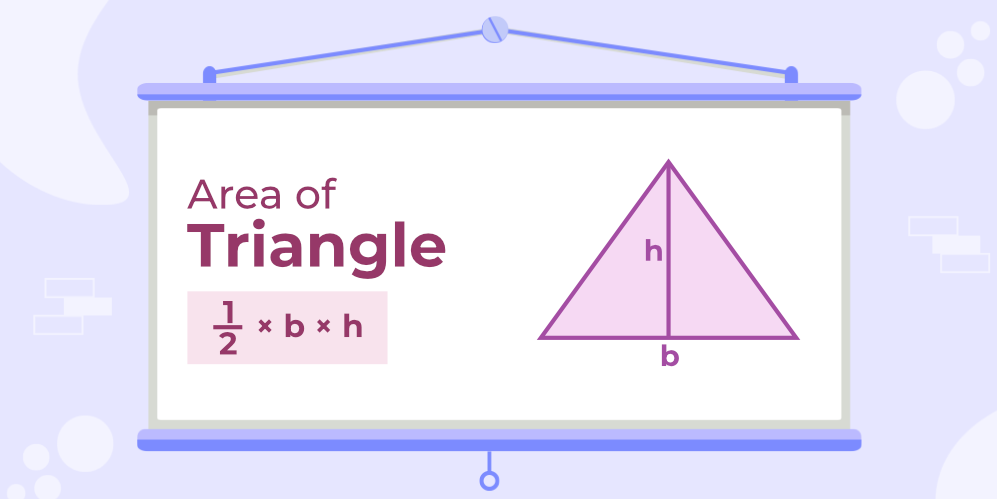Note: Base and the height of a triangle are always perpendicular to each other making a right angle(90 degrees).

### Triangle Definition

Triangle is the simplest polygon with only three sides. It is a closed figure, which can be Regular or Irregular. A regular triangle is called an Equilateral triangle. On the basis of the sides and angles of triangles, it can be categorized into various categories.

## Area of Triangle Formula

Area of triangle depends on the dimensions of the triangle, generally, the area depends on the base and height of the figure. For a triangle, the area is calculated by multiplying half the base by its height. Area of triangle is measured in square units i.e. m2, cm2, etc.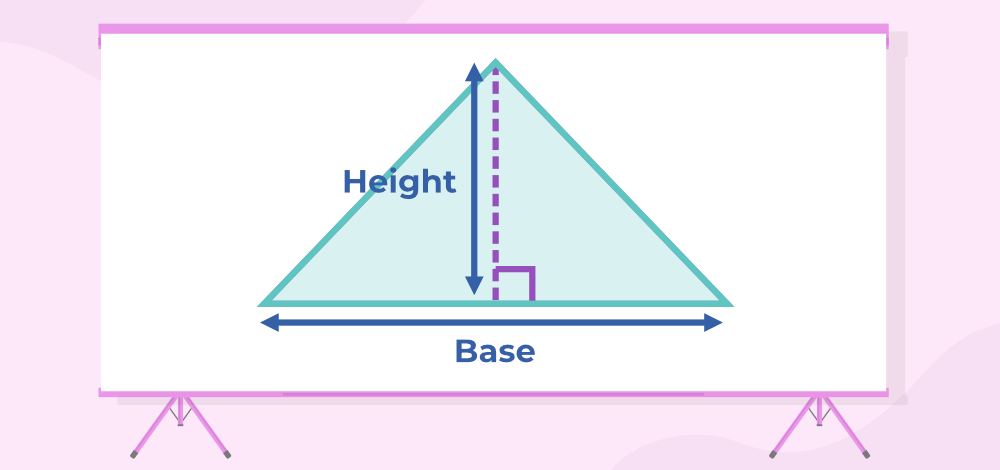Mathematically the formula is given by:

Area of triangle(A) = 1/2 × b × h

where,
b is base of the triangle
h is height of the triangle

Example: What is the area of triangle with base b = 5 m and height h = 6 m?

Solution:

Using the  general formula, Area of triangle, A = 1/2 × b × h

Area = 1/2 × 5 (m) × 6 (m)

= 5 (m) × 3 (m)

= 15 m2

## Area of Triangle With 3 Sides (Heron’s Formula)

Area of triangle with all three sides given can be found using Heron’s Formula. The application of Herons Formula includes two steps which are discussed below:

Let a triangle with sides a, b, and c are given then for finding its area,Step 1: Calculate the semi-perimeter (s) of the given triangle by

s = (a+b+c)/2

Step 2: Use Heron’s formula and find the required area.

Area = √{s(s-a)(s-b)(s-c)}

where,
s is semiperimeter
a, b, and c are sides of the given triangle.

If in a triangle all three sides are given applying the general formula is very difficult as finding height in such a case is very tricky. So we rely on Heron’s formula in such cases to find the required area.

Example: What is the area of triangle with sides of 3 cm, 4 cm, and 5 cm?

Solution:

Using Heron’s formula,

s = (a+b+c)/2

= (3+4+5)/2

= 12/2 = 6

Area = √{ s(s-a)(s-b)(s-c)}

= √{ 6(6-3)(6-4)(6-5)}

= √(6 × 3 × 2 × 1) = √(36)

= 6 cm2

Area of various types of triangles is further discussed in this article.

## Area of Triangle With Two Sides and Included Angle (SAS)

For finding the area of a triangle if the two sides of a triangle and an angle included between them are given, use the formula discussed below.

Let us take a triangle PQR, whose vertex angles are ∠P, ∠Q, and ∠R, and the sides are p,q, and r.

Now, when two sides of a triangle and the angle between them are given, then the formulas to calculate the area of a triangle is

• Area (∆PQR) = ½ qr sin P
• Area (∆PQR) = ½ pq sin R
• Area (∆PQR) = ½ rp sin Q

Example: Find the area of triangle ∆PQR, if P = 60° and q = 3, r = 2 in units.

Solution:

Given, P = 60° and q = 3, r = 2

Area (∆PQR) = ½ qr sin A

= ½ (3) (2) sin 30 (sin 60° = √3/2 )

= 3 × √3/2

=  (3√3) /2 sq.unit.

## Area of Triangle using Trigonometry

Area of triangle can be calculated using Trigonometric Ratios. Let’s learn how to calculate area of triangle using trigonometry.

Case I: When Triangle is Acute Angled

Let us assume ABC is right angled triangle and AD is perpendicular to BC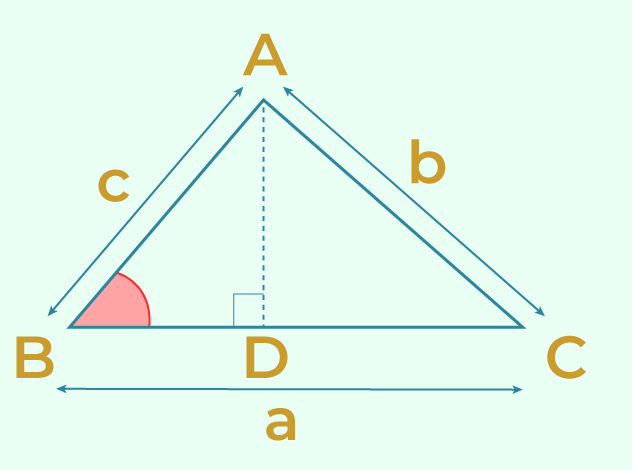In the above figure,

⇒ AD = AB Sin B = c Sin B

⇒ Area of Triangle ABC = 1/2 ⨯ Base ⨯ Height

⇒ Area of Triangle ABC = 1/2 ⨯ BC ⨯ AD

⇒ Area of Triangle ABC = 1/2 ⨯ a ⨯ c Sin B

Case II: When Triangle is Obtuse Angled

Let us assume ABC is a right angled triangle. Draw AD perpendicular to BC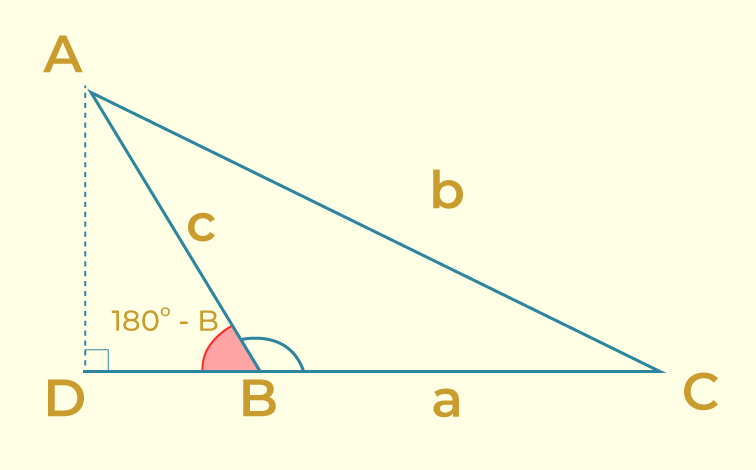In the above figure, in △ ABD, ∠ABD = (180 – B)

Hence, in △ ABD,

Sin (180 – B) = AD/AB

⇒ AD = AB ⨯ Sin B

⇒ AD = c ⨯ Sin B

Hence, area of △ ABC = 1/2 ⨯ BC ⨯ AD = 1/2 ⨯ a ⨯ c ⨯ Sin B

### Formula for Area of Triangle in Trigonometry

In the above case, we find that,

Area of Triangle = 1/2 ac Sin B when AD is perpendicular from A to BC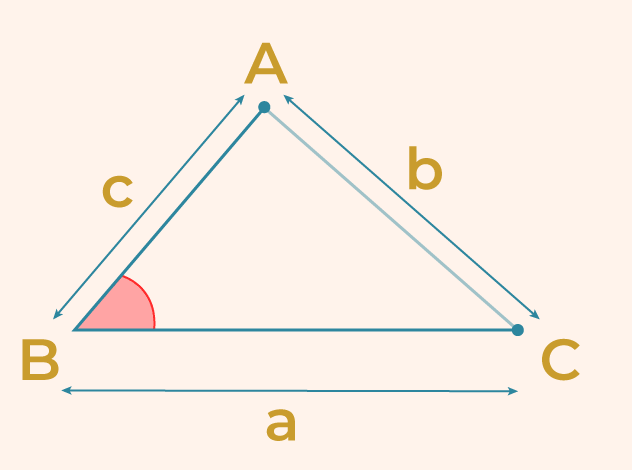If we take the altitude from different vertices the formula will be

Area of Triangle = 1/2 bc Sin A

Area of Triangle = 1/2 ab Sin C

Thus we observe that the formula for area of triangle using trigonometry is given as the half of the product of two sides and sin of the included angle.

## Area of Triangle in Determinant Form

If the coordinates of triangle ABC are given as A(x1, y1), B(x2, y2) and C(x3, y3) then Area of Triangle ABC is given in determinant form as follows:

Area of △ABC = 1/2⇒ Area of △ABC = 1/2 |x1(y2 – y3 ) + x2(y3 – y1) + x3(y1 – y2)|

## How to Find the Area of Triangle?

Area of triangle is calculated by using the following steps:

Step 1: Mark the dimensions (base, and height) of the given article.

Step 2: Use the formula for finding the area of a triangle. Area = 1/2 × (base × height)

Step 3: The area obtained in step 2 is the required area it is measured in unit2.

Thus, the area of a triangle is found using the above steps.

## Area of an Equilateral Triangle

An equilateral triangle is a triangle having all three sides equal and all three angles equal measuring 60 degrees. Suppose an equilateral triangle of sides a unit is given then the area of an equilateral triangle is given by,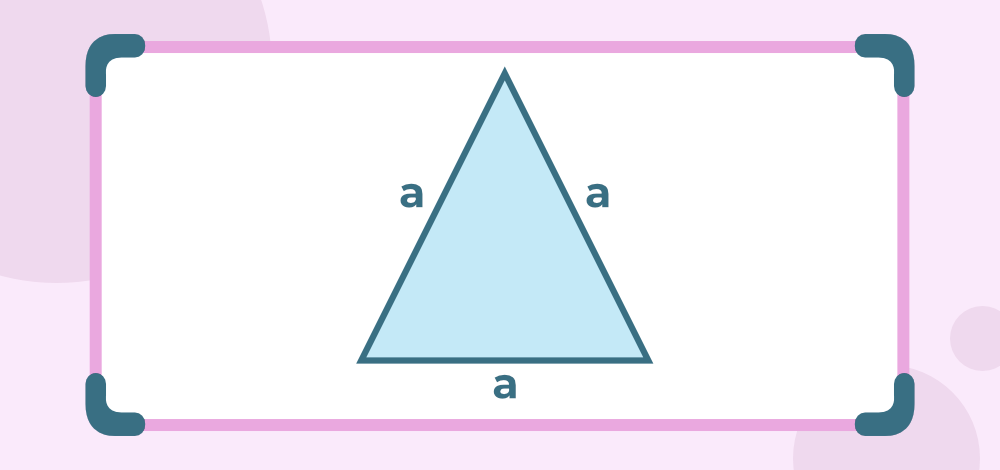Area of an Equilateral Triangle (A) = (√3)/4 × side

= (√3)/4 × a2

Example: Find the area of a triangle having side a = 8 cm

Solution:

Given,

side of the triangle (a) = 8cm

Area(A) = (√3)/4 × a2

= (√3)/4 × 82

= 16√3 cm2

## Area of an Isosceles Triangle

An isosceles triangle is a triangle having two equal sides and the angles opposite these equal sides are also equal. Suppose an isosceles triangle of common side b and another side of a, then its area is given by,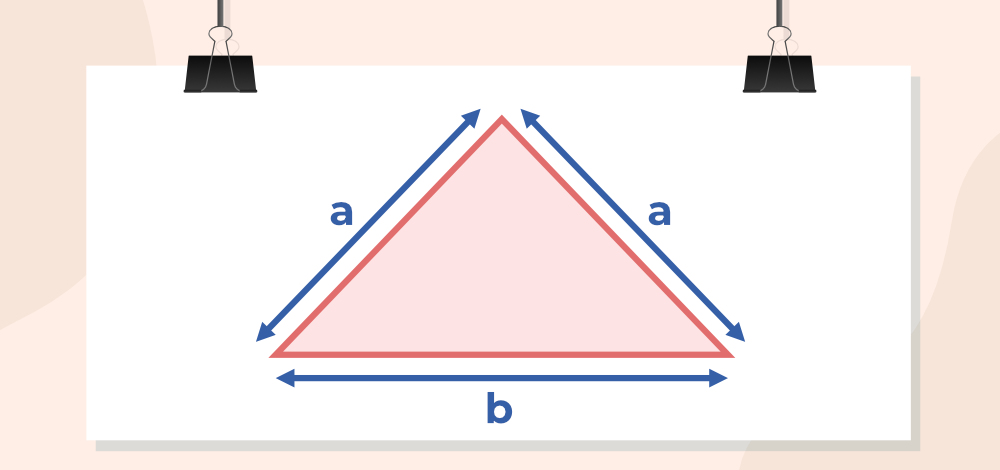Area of Isosceles triangle = ¼×b√(4a2 – b2)

Example: Find the area of an isosceles triangle having a common side a = 5 cm and other side b = 8 cm

Solution:

Given,

common side of the triangle (a) = 5cm

other side oftriangle (b) = 8cm

Area(A) = ¼×b√(4a2 – b2)

=  ¼×8√(4×52 – 82)

=  2×6 = 12 cm2

## Area of a Right Angled Triangle

A triangle that contains a right angle is considered a right-angled triangle. If all three sides in the right angled triangle are given then applying the general formula then Heron’s formula is very easy. As one of the sides in the right-angle triangle behaves as the base and the other as height. In the figure given below, a is the base of the triangle, and c is its height of it. So applying the general formula is easy here,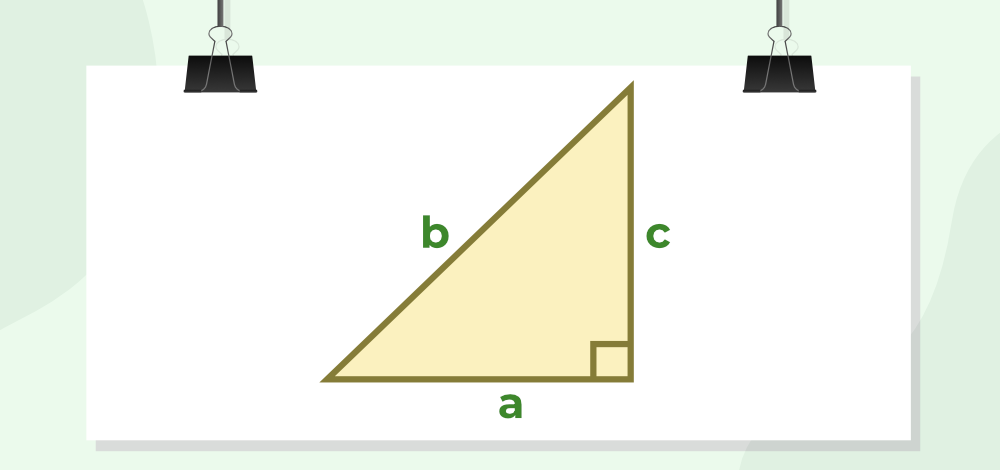Area of a Right Triangle (A) = 1/2 × a × c

where,
a is base of the triangle
c is height of the triangle

Example: Find the area of triangle having a base of a = 8 cm and a height c = 5 cm.

Solution:

Given

Base of the triangle (a) = 8cm

Height of the triangle (c) = 5cm

We have,

Area(A) = 1/2 × a × c

= 1/2 × 8 × 5

= 20 cm2

## What is the Perimeter of a Triangle?

Perimeter of the triangle is defined as the total length of the boundary of the triangle. For example, if a triangle has three sides of length a m, b m, and c m then its perimeter is (a + b + c) m.

Let a triangle of the triangle ABC is taken then,

Perimeter of △ABC = AB + BC + CA

Example: The triangle park has three sides of 15 m, 18 m, and 20 m. If a man runs along the boundary of the park 5 times find the distance travelled by him.

The side of the park is,

• a = 15 m
• b = 18 m
• c = 20 m

Perimeter of the Park = a + b + c

= 15 + 18 + 20 = 53 m

The distance travelled by the man = 5 × 53

= 165 m

Related Articles,

## Solved Examples on Area of Triangle

Example 1: Find the area of triangle having a base of 12 cm and a height of 4 cm.

Solution:

Given

Base of the triangle = 12cm’

Height of the triangle = 4cm

We have,

Area(A) = 1/2 × b × h

= 1/2 × 12 × 4

= 24cm2

Example 2: What is the area of triangle with sides of 8 cm, 6 cm, and 10 cm (using Heron’s Formula)?

Solution:

Using Heron’s formula,

s = (a+b+c)/2

= (8+6+10)/2

= 24/2 = 12

Area = √{ s(s-a)(s-b)(s-c)}

= √{ 12(12-8)(12-6)(12-10)}

= √(12×4×6×2) = √(576)

= 24 cm2

Example 3: Find the area of a right-angled triangle having base a = 5 cm and height c = 3 cm.

Solution:

Given

Base of the triangle (a) = 5 cm

Height of the triangle (c) = 3 cm

We have,

Area(A) = 1/2 × a × c

= 1/2 × 5 × 3

= 7.5 cm2

Example 4: Find the area of an equilateral triangle having side a = 6 cm

Solution:

Given,

side of the triangle (a) = 6 cm

Area(A) = (√3)/4 × a2

= (√3)/4 × 62

= 9√3 cm2

Example 5: Find the area of triangle having a base of 15cm and a height of 5cm.

Solution:

The base of the triangle=15 cm

Height of the triangle=5 cm

We have,

Area(A) = 1/2 × b × h

= 1/2 × 15 × 5

= 37.5 cm2

## Practice Problems on Area of Triangle

1. Find the area of triangle with a base of 8 inches and a height of 5 inches.

2. Calculate the area of an equilateral triangle with a side length of 6 centimeters.

3. Given a right triangle with one leg measuring 10 meters and the other leg measuring 24 meters, what is the area of triangle?

4. Determine the area of an isosceles triangle with a base of 12 feet and each of the congruent sides measuring 9 feet.

5. A triangle has a base of 15 inches and an area of 60 square inches. Find its height.

## FAQs on Area of Triangle

### 1. What is the Area of triangle?

The region enclosed by the boundary of the triangle i.e. area occupied by the perimeter of the triangle is termed the area of the Triangle.

### 2. How to calculate the Area of Triangle?

Area of the Triangle can be calculated using the formula,

Area = 1/2 × b × h

where
b is the base of triangle
h is the height of triangle

### 3. What is the Area of Triangle when Vertices are given?

When all three vertices of the triangle A(x1, y1), B(x2, y2) and C(x3, y3) are given then its area is calculated using the formula,

Area = 1/2 × [x1(y2 – y3) + x2(y3 – y1) + x3(y1 – y2)]

### 4. What is the Area of Triangle when three sides are given?

If all the three sides of Triangle are given then its area is calculated using the Herons Formula. Let the side of triangle be a, b, and c then its area is

Area = √{ s(s-a)(s-b)(s-c)}

where,
s is semi-perimeter

### 5. What is the Area of Triangle in Vector form?

If a triangle is formed by two vectors u, and v then its area is given by half of magnitude of product of the given vectors i.e.

Area = 1/2|×|

### 6. What are the Formulas for Area of Triangle?

Area formulas for triangles:

1. For a Right Triangle: Area = (1/2) ⨯ base ⨯ height

2. For a Triangle with Given Base and Height: Area = (1/2) ⨯ base ⨯ height

3. For a Triangle with Heron’s Formula: Area = √(s ⨯ (s – a) ⨯ (s – b) ⨯ (s – c)), where “s” is the semi-perimeter.

### 7. How to Find Area of Triangle?

To find the area of a triangle:

1. For a right triangle: Area = (1/2) ⨯ base ⨯ height.

2. For a triangle with a known base and height: Area = (1/2) ⨯ base ⨯ height

3. For any triangle with all three side lengths known: Use Heron’s formula, Area = √(s ⨯ (s – a) ⨯ (s – b) ⨯ (s – c)), where “s” is the semi-perimeter, and “a,” “b,” and “c” are the side lengths.

Whether you're preparing for your first job interview or aiming to upskill in this ever-evolving tech landscape, GeeksforGeeks Courses are your key to success. We provide top-quality content at affordable prices, all geared towards accelerating your growth in a time-bound manner. Join the millions we've already empowered, and we're here to do the same for you. Don't miss out - check it out now!

Previous
Next